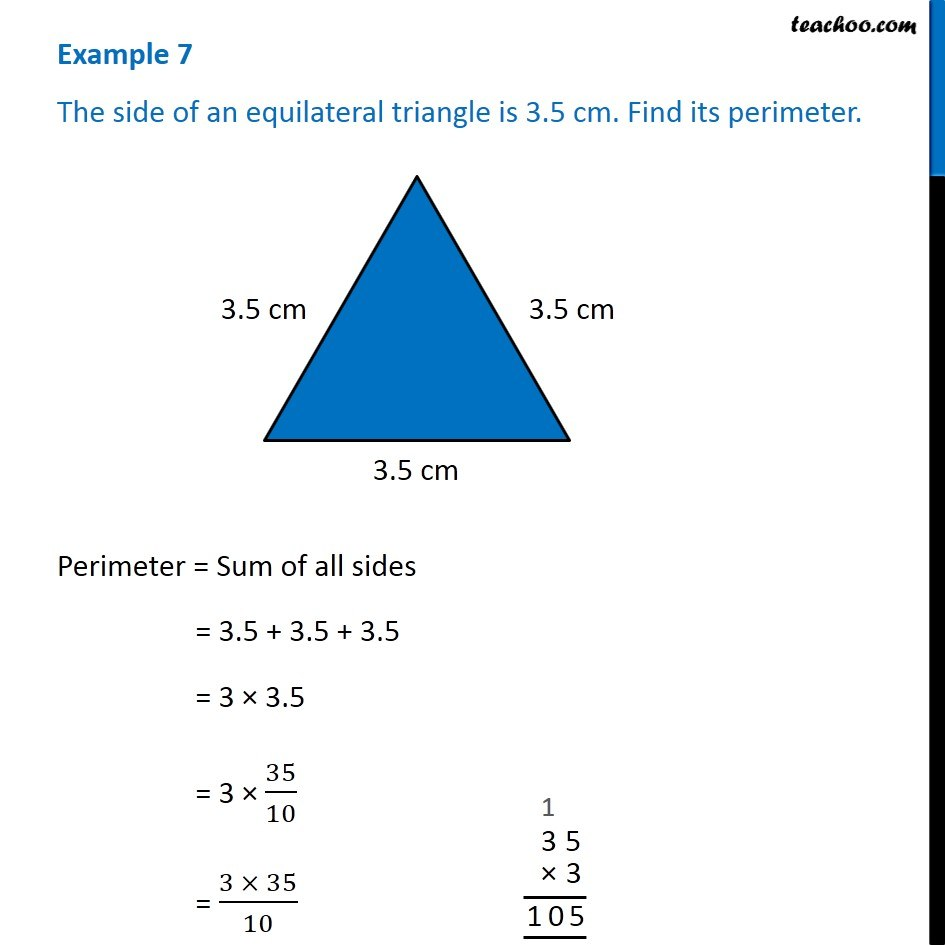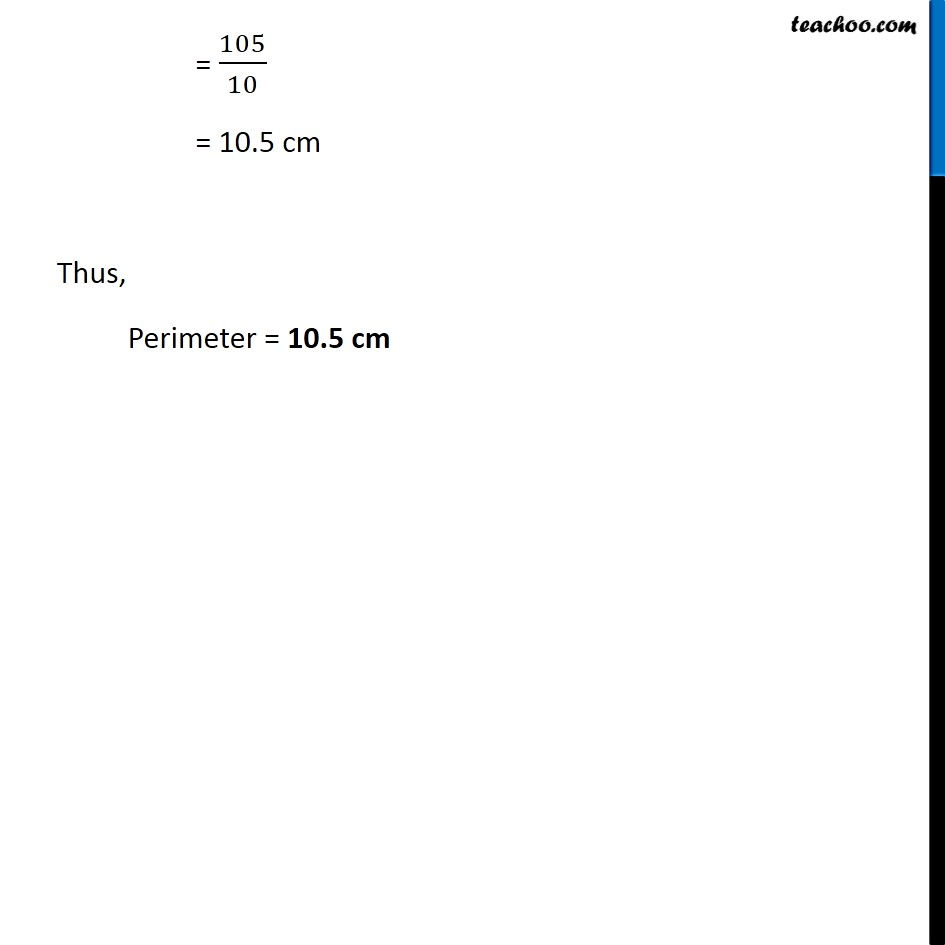1. Chapter 2 Class 7 Fractions and Decimals
2. Concept wise
3. Multiplication of Decimals - Statement Questions

Transcript

Example 7 The side of an equilateral triangle is 3.5 cm. Find its perimeter. Perimeter = Sum of all sides = 3.5 + 3.5 + 3.5 = 3 × 3.5 = 3 × 35/10 = (3 × 35)/10 = 105/10 = 10.5 cm Thus, Perimeter = 10.5 cm

Multiplication of Decimals - Statement Questions